## BREAK NEWS# Kepler’s law of planetary motion and gravitational constant## Kepler’s Law of Planetary Motion

1. Planets revolve in elliptical orbits having the sun at one focus
2. Each planet revolve in such a way that the imaginary line joining it to the sun sweeps out equal areas in equal times
3. The squares of the periods of revolution of the planets are proportional to the cubes of their mean distances from the sun

### Newton’s law of gravitation

The force of attraction between two bodies is directly proportional to the product of their masses and inversely proportional to the squares of their distance apart.Consider two bodies of mass M and m with a distance r apartProof of Kepler’s 3rd law

Consider a planet of mass m moves with speed v in a circle of radius r around the sun of mass M.### Mass and density of the earth

For a body of mass, m, on the earth’s surface, the force of gravity acting on it is given by F = mg

The earth of mass M and radius r is assumed to be spherical and uniform and thus its mass concentrated at its center. The force of attraction on the earth on the body is given by### Mass of the sun

Consider the earth of mass m revolving around the sun of Mass, M at a distance r from the sun

The centripetal force on the earthT = 1year  = 365 x 60 x 60 = 31, 536, 000s,

G =  6.67 x 10-11Nm2kg,

r  =  150 x 106km= 1.5 x 1011m

M =  = 2 x 1030kg

Assumptions

• The sun is stationary
• The earth is spherical

Example 1

State the effect of frictional forces on the motion of an earth satellite against distance from the earth’s center.

Solution

The mechanical energy of a satellite decrease, potential energy decrease, kinetic energy increases. The velocity of the satellite increase while the radius of the orbit decrease and satellite may burn if no precaution is taken.

A graph showing the variation of energy of a satellite against distance from the earth’s center.Escape velocity

This is the vertical velocity that should be given to a body at the surface of a planet so that it just escapes from the gravitational attraction of the planet.

Derivation

Consider a body or a rocket of mass, m, fired from the earth’s surface such that it escapes from the gravitational influence of the earth. However, it requires a certain velocity to escape.At the surfaceThis shows that the escape velocity is independent of the mass of the body.

Example 2

Explain why the moon has no atmospheres yet the sun has

Solution

This is because the gravitational attraction at the moon is too weak to hold the gases due to its small mass and as a result, the gases escape leaving the moon without atmospheres.

### Variation of acceleration due to gravity, g, with latitude

The acceleration due to gravity, g, varies over the earth because the earth is elliptical, with the polar radius, b = 6.357 x 106m and the equatorial radius, a = 6.378 x 107m, hence as one moves from the equator to the poles the distance of the point on the surface of the earth from the center of the earth decreases. Hence the acceleration due to gravity or attraction of the body towards the center increases. (note that force of attraction due to gravity at a place inversely proportional to the distance  of the point from the center of the earth squared)

The latitude of a point is the angle θ between the equatorial plane and the line joining that point to the center of the earth. The latitude of the equator is 00 and that of the pole is 900.

Consider a body of mass, m, at point P with latitude θ as shown on the surface of the earth and gθ be the acceleration due to gravity at P.Due to rotation motions of the earth about its axis P experiences a centripetal force given by mrω2

Resolving the centripetal force into two rectangular components, its component along the radius of the earth = mrω2cosθ

Also, the body is acted on by two forces; its weight acting towards the center of the earth and the component, mrω2cosθ acting radially outwards.

The difference between the two forces is the weight of the body at that point at that point

mgθ = mg – mrω2cosθ ………………….. (i)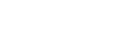………………………….. (ii)

Substituting equation (ii) in (i)

mgθ = mg – m(Rcosθ)ω2cosθ

gθ = g – Rω2cos2θ

This is the expression for acceleration due to gravity at point P on the earth’s surface having latitude θ.

At equator θ = 00, hence gθ is minimum. At the pole θ = 900, hence, gθ is maximum.

Example 3

What is the weight of a body of mass 1000kg on the earth at

• Equator
• Pole
• Latitude of 300 (Radius of the earth, R = 6400km, acceleration due to gravity, g = 9.81ms-2)

Solution

Period of the earth, T = 24 x 60 x 60s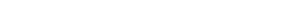The  weight of a body Wθ = latitude θis given by

Wθ = m(g – Rω2cos2θ)

• At the equator θ =00

W0 = 1000[9.81 – 6.4 x 106(7. 273 x10-5)2cos20]

=9776.15N

• At the pole θ = 900

W0 = 1000[9.81 – 6.4 x 106(7. 273 x10-5)2cos290]

=9810N

• At the latitude where θ = 300

W0 = 1000[9.81 – 6.4 x 106(7. 273 x10-5)2cos230]

=9784.61N

### Variation of acceleration to gravity due to depthWhen the body is a distance, d, below the earth’s surface, the acceleration due to gravity, gd is given byThe expression shows that acceleration due to gravity reduces as depth, d, increases toward the earth’s center. At the center d = R, hence acceleration due to gravity = 0.

### Variation of acceleration due to gravity with altitudeWe have

GM = R2g………………. (i)

Gm = (R +h)2gh ……………(ii)

From (i) and (ii)

R2g =(R +h)2ghThe expression shows that acceleration due to gravity decreases as h increases.

A graph of variation of acceleration due to gravity against the distance from earth’s center.Example 1

At what height will a man’s weight become half his weight on the surface of the earth? Take the radius of the earth as R.

Solutionbut r = (R + h)

R + h = R  = 1.414R

H = 0.414R

### Determining gravitational constant(i) Two equal lead spheres A and B each of mass, m, are attached to end of a bar AB of length, L.

(ii) The bar AB is suspended from a ceiling.

(iii) Large spheres P and Q are brought towards A and B respectively from the opposite side

(iv) Large spheres P and Q altered small spheres A and B respectively by equal and opposite gravitational forces give         rise to gravitational torque, F, which in turn twists the suspended through angle θ.

(v) A resting torque of the wire opposes the twisting of the wire from the equilibrium position

ThenWhere

d = distance between the center of A and P or B and Q.

C = The twisting couple per unit twist (θ = 1)

Artificial satellite

It is a huge body that moves around a planet is an orbit due to the force of gravitation attraction. Satellite can be launched from the earth’s surface to circle the earth. The are kept in their orbit the gravitational attraction of the earth.

Consider a satellite of mass, m, moving around the earth of mass M in an orbit of radius, r.## Parking orbital

A parking orbit is a path in space of a satellite which makes it appear to be in the same position relative to the observer at a point on the earth.

An object in a parking orbit has a period equal to the period of the earth’s rotation about its axis. The direction of motion of the object in a parking orbit is in the same sense as the rotation of the earth about its axis.

The angular velocity of an object in its orbit is equal to that of the earth as it rotates about its axis. An object in a parking orbit is directly above the equator

Uses of parking orbits

Used in telecommunication

Many geostationary satellites are required for efficient broadcasting which makes very expensive.

Communication can only occur provided there is no obstruction between the transmitter and the receiver.

Gravitational Potential energy

This is the work done in moving a body of mass m from infinity to a point in the earth’s gravitational field.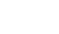w = F∆r

Total work done in moving a mass m from infinity to a point from the earth of mass, M is given by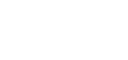### Kinetic energy

Consider a body of mass, m, moving around the earth of mass, M, with velocity v.For this reason, the moon is very cold. The sum has atmosphere because of the stronger gravitational attraction caused by its mass and a much higher escape velocity

WeightlessnessLet g’ = acceleration due to gravity at a certain height

R = reaction of the spacecraft in contact with an astronaut.

Consider a body moving in a spacecraft at a particular height of the orbit.

R = mg’

ma = mg’-R

If g’ = a

When mg’ = ma

then R = 0

Therefore the body becomes weightless

Example 2

A body of mass 15kg is moved from the earth’s surface to a point 1.8 x 106m above the earth. if the radius of the earth is 6.4 x 106 and the mass of 6.0 x 1024kg, calculate the work done in taking the body to that point.

Solution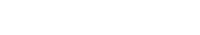a = 6.4 x 106m

b = 6.4 x 106 + 1.8 x 106 = 9.2 x 106m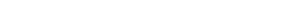Example 3

(a) With the aid of a diagram, describe an experiment to determine universal constant, G.

(b) If the moon moves around the earth in a circular orbit of radius = 4.0 x 108m and takes exactly 27.3days to go              round once. Calculate the value of acceleration due to gravity g at the earth’s surface.

SolutionExample 4

(a) Show how to estimate the mass of the sun if the period and orbital radius of one of its planet is known. The                 gravitational potential u1 at the surface of a planet of mass M and radius R is given by where G is the                             gravitational constant.

Derive an expression for the lowest velocity, v1, which an object of mass, M, must have of the planet if it is to escape from the planet.

(b) Communication satellites orbit, the earth in synchronous orbits. Calculate the height of the communication satellite above the earth.

SolutionCATEGORIES